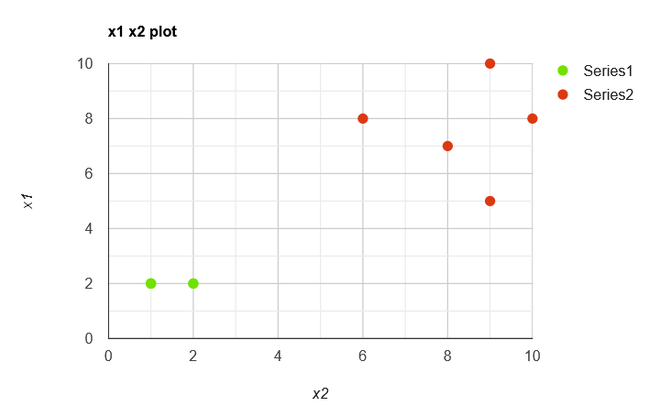Problem solving on scatter matrix

• Difficulty Level : Expert
• Last Updated : 10 Sep, 2021

Prerequisite : Scatter Plot Matrix

We calculate Sw  ( within class scatter matrix ) and SB ( between class scatter matrix )  for the available data points.

SW : To minimize variability within a class, inner class scatter.
SB :  To increase between class variability, between class scatter.scatter plot of the points

X1 = (y1, y2) ={ (2,2), (1,2), (1,2), (1,2), (2,2) }
X2 = (y1,y2) ={ (9, 10), (6,8), (9,5), (8,7), (10,8) }

Within class scatter matrix :Si is the class specific covariance matrix.
mi is the mean of indivisual class

Mean computation :

We calculate the mean for each of the points present in the class. Here mean is the total sum of observations divided by the number of observations, we require this mean fot calculating the covariance of the matrix.Covariance matrix computation :
We subtract the mean value from each of the observation and then we calculate the average after performing the matrix multiplication with the transpose of the matrix.

Class specific covariance for the first class :Averaging values from 1,2,3,4 and 5.
We calculate the sum of all the values for each element in the matrix for S1 and divide by the number of the observation which in the present computation is 5.Therefore S1 is :Class specific covariance for the second class :Averaging values from 1,2,3,4 and 5
we calculate the sum of all the values for each element in the matrix for S2 and divide by the number of the observation which in the present computation is 5.Therefore S2 is :Within class scatter matrix Sw :

SW = S1 + S2Between class scatter matrix SB  :Total scatter matrix :

ST = SB + SWTherefore we have calculated between class scatter matrix and within class scatter matrix for the available data points.

We make use of these computations in feature extraction , where the main goal is to increase the distance between the class in the projection of points and decrease the distance between  the points within the class in the projection. Here we aim at generating data projection at the required dimension.

My Personal Notes arrow_drop_up